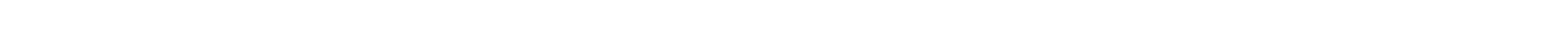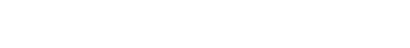## Swift Tutorial

If Statement Switch statement Loops Decision making in SwiftThe programmer needs a number of assessment circumstances for the program in this sort of declaration. The declaration(s) is performed if the situation is true and, if it is wrong, an alternative declaration or set of statements is performed. In its programming, Swift offers the following kinds of decisions. These are as follows : If Statement: The first and most frequent language in all programming is IF It regulates the program flow by allowing the user. This conditional declaration only operates if the condition is true. Syntaxif boolean_expression {  /* statement(s) will execute if the boolean expression is true */  } Example import Cocoa  var a1:Int=100;  if a1 >50{ /* if the condition is true then print */  println("1st Variable is greater than 50"); }  println("The value of variable is \(a1)")If … else Statement: If statement, an optional other statements will follow that executes if the Boolean expression is false. Syntaxif boolean_expression { // statement(s) will execute if the boolean expression is true } else { //  otherwise statement(s) will execute if the boolean expression is false } Example var x:Int=100; if x <50{ println("Value is less than 50"); }else{ println("Value is not less than 50"); } println("Value of variable is \(x)");Else if Statement: An if statement can be followed by an optional else if...else statement, which is very useful to test various conditions using single if...else if statement. Syntaxif boolean_expression_1 { } else if boolean_expression_2 { . } else if boolean_expression_n { ...... } else { } Nested if Statement: You can use one if or else if statement inside another if or else if statement(s). Syntaxif boolean_expression_1 { // Executes when the boolean expression 1 is true if boolean_expression_2 { // Executes when the boolean expression 2 is true } }Switch Statement: Another type of a conditional statement, the switch statement, can be used to regulate the flow of your code. Here a different case or block is executed. As the case value matches the block of the statement following the case gets executed. Syntaxswitch expression { case expression1 : statement(s) fallthrough // optional case expression2, expression3 : statement(s) fallthrough //optional default:// Optional statement(s); } Here, if you do not use a fallthrough statement, then after executing the matching case statement the program will come out of the switch statement. Thus the declaration of fallthrough is similarly essential for Switch declarations in Swift language. Exampleimport Cocoa var value = 30 switch index { case 70: println("Value of index is 70") fallthrough case 10,30 : println("the value is either 10 or 30") fallthrough case 50: println("The value is 50") default : println("Wrong Case Input") }Output the value is either 20 or 30 The value is 50 Loops Looping, also known as iteration is used to repeat a particular block / section of code(s) in a program. This decreases the duties of repeatedly having to write the same thing. This is a flowchart that shows how loop statements function:The following types of loops can be used in the Swift programming language. Please find below details of all loops : The for-in loop: The for-condition-increment loop is functionally the same as the 'for' loop in C. The loop comprises an initialization stage, a test, an increase and a set of declarations that are carried out for each loop iteration. Syntax for index in var {     statement(s)  }ExampleThis iterates through a range of numbers in the below example, the loop index variable i is given each time through the loop, by the next number within the range: for i in 3...6{ print(i) }The while loop: In the while loop, the condition is tested prior to the body of the loop and only if the condition is correct the procedure is performed.The following is the overall format: Syntax while condition { statements }Statement(s) can be a single declaration or a statement block here. The situation could be any term. The circuit is iterated while the state is true. The control of the program moves instantly after the loop when the situation is incorrect. Example var i=0  while(i <9){  print(i)  i++  } Repeat while loop: The termination condition at the end of the loop is tested by repeated loops rather than at the beginning. This implies that the statements are made at least once in the body of the loop. Continues loop execution happens until the condition evaluates to false.The flow diagram is as follows:The following is the format for repeated loops. Syntax: repeat { statements } while condition Example var i = 0 repeat { print (i) i++ } while (i < 5)Jump statements of SwiftThe Continue statement: In Swift's continuing statement, the loop is instructed to stop what is being done and start through the loop again at the start of the next iteration. Example var value= 8  do{  value = value+1  if(value==10){    continue  }     println("Value is \(value)")  }whilevalue< 12The Break statement:The declaration of break is used inside a circuit. The loop is finished immediately and the program control resumes after the loop at the next declaration. Example var value = 8  do{  value = value+1  if(value==10){      break  }     println("Value is \(value)")  }whilevalue<12Summary:This module helped us in understanding control flow in swift. This flow is needed whenever we have to take any decision .Decision making mechanisms allow the programmer to define one or more conditions to be checked or tested by the program, together with a statement or statements to be executed if the condition is determined to be true, and optionally, other statements to be executed if the condition is found to be false.

# Control Flow

• If Statement
• Switch statement
• Loops

### Decision making in Swift

The programmer needs a number of assessment circumstances for the program in this sort of declaration. The declaration(s) is performed if the situation is true and, if it is wrong, an alternative declaration or set of statements is performed.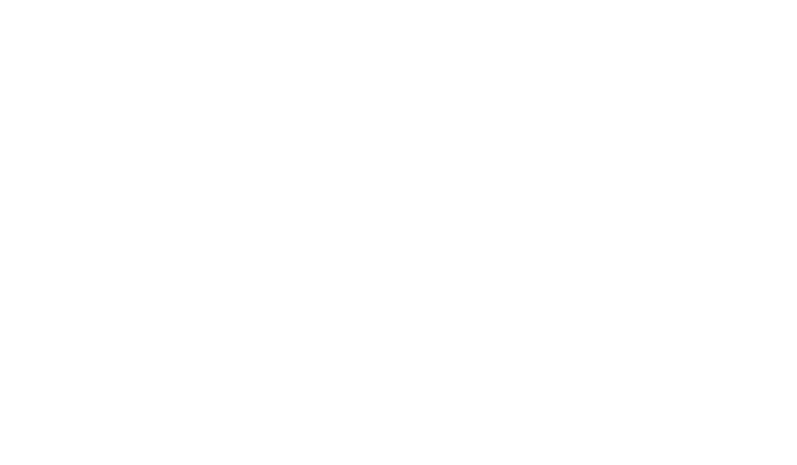In its programming, Swift offers the following kinds of decisions. These are as follows :

• If Statement: The first and most frequent language in all programming is IF It regulates the program flow by allowing the user. This conditional declaration only operates if the condition is true.

Syntax

if boolean_expression {
/* statement(s) will execute if the boolean expression is true */
}

Example

import Cocoa
var a1:Int=100;
if a1 >50{

/* if the condition is true then print */
println("1st Variable is greater than 50");
}
println("The value of variable is \(a1)")
• If … else Statement: If statement, an optional other statements will follow that executes if the Boolean expression is false.

Syntax

if boolean_expression {

// statement(s) will execute if the boolean expression is true

} else {

//  otherwise statement(s) will execute if the boolean expression is false
}

Example

var x:Int=100;
if x <50{
println("Value is less than 50");
}else{
println("Value is not less than 50");
}
println("Value of variable is \(x)");
• Else if Statement: An if statement can be followed by an optional else if...else statement, which is very useful to test various conditions using single if...else if statement.

Syntax

if boolean_expression_1 {

else if boolean_expression_2 {

.

else if boolean_expression_n {

......

else {

}

• Nested if Statement: You can use one if or else if statement inside another if or else if statement(s).

Syntax

if boolean_expression_1 {
// Executes when the boolean expression 1 is true
if boolean_expression_2 {
// Executes when the boolean expression 2 is true
}
}
• Switch Statement: Another type of a conditional statement, the switch statement, can be used to regulate the flow of your code. Here a different case or block is executed. As the case value matches the block of the statement following the case gets executed.

Syntax

switch expression {
case expression1 :
statement(s)
fallthrough // optional
case expression2, expression3 :
statement(s)
fallthrough //optional
default:// Optional
statement(s);
} 

Here, if you do not use a fallthrough statement, then after executing the matching case statement the program will come out of the switch statement. Thus the declaration of fallthrough is similarly essential for Switch declarations in Swift language.

Example

import Cocoa
var value = 30
switch index {
case 70:
println("Value of index is 70")
fallthrough
case 10,30 :
println("the value is either 10 or 30")
fallthrough
case 50:
println("The value is 50")
default :
println("Wrong Case Input")
}
Output

the value is either 20 or 30
The value is 50 

### Loops

Looping, also known as iteration is used to repeat a particular block / section of code(s) in a program. This decreases the duties of repeatedly having to write the same thing. This is a flowchart that shows how loop statements function: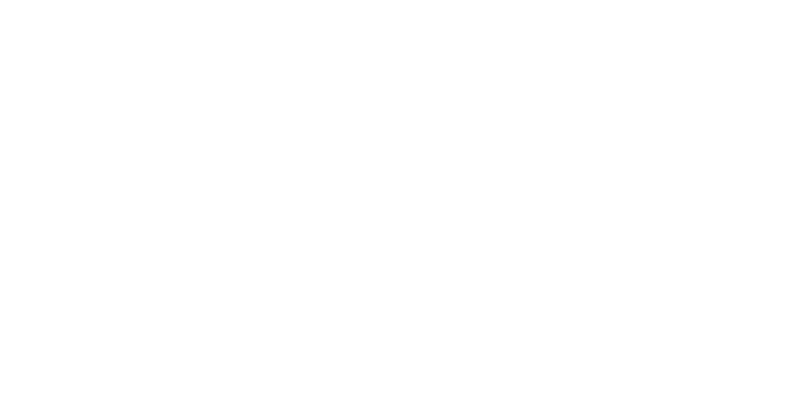The following types of loops can be used in the Swift programming language. Please find below details of all loops :

The for-in loop: The for-condition-increment loop is functionally the same as the 'for' loop in C. The loop comprises an initialization stage, a test, an increase and a set of declarations that are carried out for each loop iteration.

Syntax

for index in var {
statement(s)
}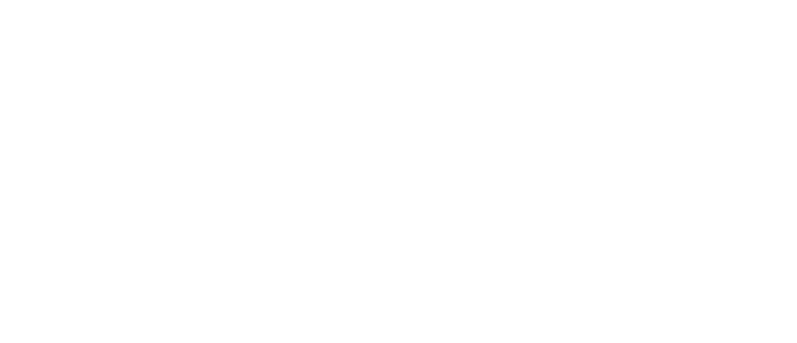Example

This iterates through a range of numbers in the below example, the loop index variable i is given each time through the loop, by the next number within the range:

for i in 3...6{
print(i)
}

The while loop: In the while loop, the condition is tested prior to the body of the loop and only if the condition is correct the procedure is performed.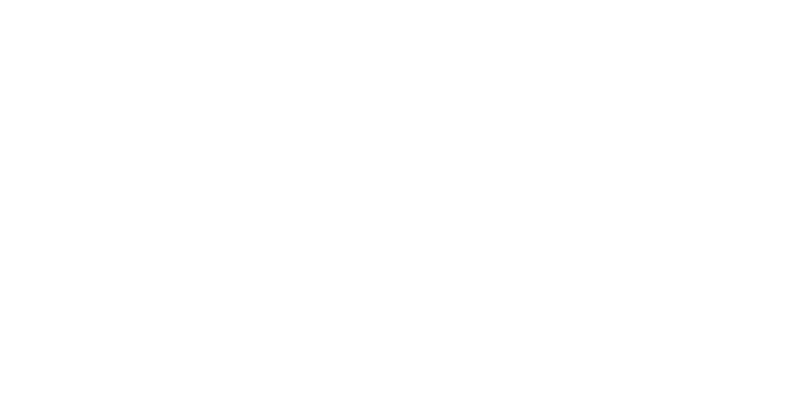The following is the overall format:

Syntax
while condition {
statements
}

Statement(s) can be a single declaration or a statement block here. The situation could be any term. The circuit is iterated while the state is true. The control of the program moves instantly after the loop when the situation is incorrect.

Example

var i=0
while(i <9){
print(i)
i++
} 

Repeat while loop: The termination condition at the end of the loop is tested by repeated loops rather than at the beginning. This implies that the statements are made at least once in the body of the loop. Continues loop execution happens until the condition evaluates to false.The flow diagram is as follows: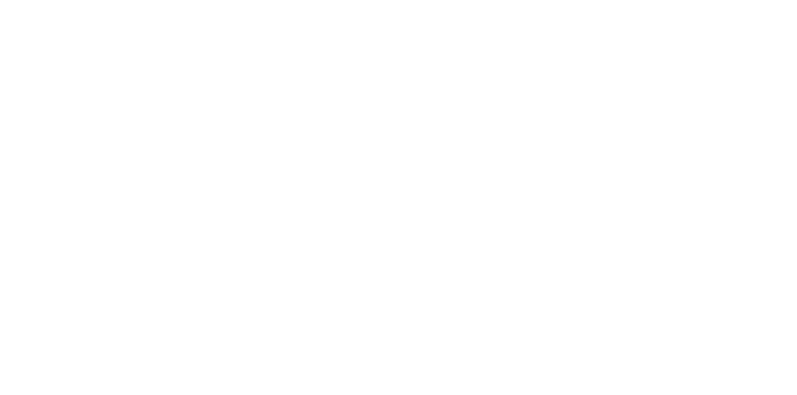The following is the format for repeated loops.

Syntax:

repeat {
statements
} while condition

Example

var i = 0
repeat {
print (i)
i++
} while (i < 5)

### Jump statements of Swift

The Continue statement: In Swift's continuing statement, the loop is instructed to stop what is being done and start through the loop again at the start of the next iteration.

Example

var value= 8
do{
value = value+1
if(value==10){
continue
}
println("Value is \(value)")
}whilevalue< 12

The Break statement:The declaration of break is used inside a circuit. The loop is finished immediately and the program control resumes after the loop at the next declaration.

Example

var value = 8
do{
value = value+1
if(value==10){
break
}
println("Value is \(value)")
}whilevalue<12

Summary:

This module helped us in understanding control flow in swift. This flow is needed whenever we have to take any decision .Decision making mechanisms allow the programmer to define one or more conditions to be checked or tested by the program, together with a statement or statements to be executed if the condition is determined to be true, and optionally, other statements to be executed if the condition is found to be false.#### Arlin Burns

Is this included in csm training and certification or sold separately?#### Sneha Agrawal

I am interested in advanced level training of SWIFT and coding.

R Programming

## C# Tutorial

C# is an object-oriented programming developed by Microsoft that uses the .Net Framework. It utilizes the Common Language Interface (CLI) that describes the executable code as well as the runtime environment. C# can be used for various applications such as web applications, distributed applications, database applications, window applications etc.For greater understanding of this tutorial, a basic knowledge of object-oriented languages such as C++, Java etc. would be beneficial.
22602
C# Tutorial

C# is an object-oriented programming developed by Microsoft that uses ...